• 项目设计：最小权顶点覆盖问题 给定一个赋权无向图 G=(V,E)，每个顶点 v V ∈ 都有一个权值 w(v)。如果 U 包含于 V， 且对于 ， 且对于(u,v) E ∈ 有 u U ∈ 且 v V ∈ -U，则有 v K. ∈ 如：U = {1}, 若有边（1...
• 最小权顶点覆盖问题在实际决策中应用广泛，但顶点上的权值在实际应用中通常代表费用、成本等，在很多情况下是不确定的。关注了最小权顶点覆盖问题中的模糊不确定性，对模糊环境下的最小权顶点覆盖问题进行了研究。...模糊变量 可信性理论 模糊模拟 遗传算法
• 6-3 最小权顶点覆盖问题 问题描述 给定一个赋权无向图 G=(V,E)，每个顶点 v∈V 都有一个权值 w(v)。如果 U⊆VU⊆VU\subseteq V，且对任意(u,v)∈E 有 u∈U 或 v∈U，就称 U 为图 G 的一个顶点覆盖。G 的最小权...
6-3 最小权顶点覆盖问题

问题描述
给定一个赋权无向图 G=(V,E)，每个顶点 v∈V 都有一个权值 w(v)。如果

U⊆V

U

⊆

V

$U\subseteq V$，且对任意(u,v)∈E 有 u∈U 或 v∈U，就称 U 为图 G 的一个顶点覆盖。G 的最小权顶点覆盖是指 G 中所含顶点权之和最小的顶点覆盖。
对于给定的无向图 G，设计一个优先队列式分支限界法，计算 G 的最小权顶点覆盖。
数据输入：  第 1 行有 2 个正整数 n 和 m，表示给定的图 G 有 n 个顶点和 m 条边，顶点编号为 1，2，…，n。第 2 行有 n 个正整数表示 n 个顶点的权。接下来 的 m 行中，每行有 2 个正整数 u,v，表示图 G 的一条边(u,v)。

Java
package Chapter6FenZhiXianJieFa;

import java.util.PriorityQueue;
import java.util.Queue;
import java.util.Scanner;

public class ZuiXiaoQuanDingDianFuGai {

private static class HeapNode implements Comparable{
int i,cn;
int[] x,c;

public int compareTo(Object o){
HeapNode heapNode = (HeapNode) o;
int result = Integer.compare(cn, heapNode.cn);

return result;
}
}

private static int u,v;
private static int n,e;
private static int[][] a;
private static int[] p;

public static void main(String[] args){
Scanner input = new Scanner(System.in);

while (true){
n = input.nextInt();
e = input.nextInt();

a = new int[n+1][n+1];
p = new int[n+1];

for(int i=0; i<=n; i++)
for(int j=0; j<=n; j++)
a[i][j] = 0;

for(int i=1; i<=n; i++)
p[i] = input.nextInt();

for(int i=1; i<=e; i++){
u = input.nextInt();
v = input.nextInt();
a[u][v] = 1;
a[v][u] = 1;
}

System.out.println(minCover(a,p,n));
for(int i=1; i<=n; i++)
System.out.print(p[i]+" ");
}
}

private static class VC{
int[][] a;
int[] w,bestx;
int n,bestn;

private void BBVC(){
Queue<HeapNode> H = new PriorityQueue<>(100000);
HeapNode E = new HeapNode();
E.x = new int[n+1];
E.c = new int[n+1];
for(int j=1; j<=n; j++) {E.x[j]=E.c[j]=0;}
int i=1,cn=0;
while (true){
if(i > n){
if(cover1(E)){
for(int j=1; j<=n; j++) bestx[j]=E.x[j];
bestn = cn;
break;
}
}else{
}
if(H.size() == 0) break;
E = H.poll();
cn = E.cn;
i = E.i+1;
}
}

//        private boolean cover(HeapNode E){
//            for(int j=1; j<=n; j++)
//                if(E.x[j]==0 && E.c[j]==0) return false;
//
//            return true;
//        }

private boolean cover1(HeapNode E){
for(int i=1; i<=n; i++)
for(int j=1; j<=n; j++)
if(a[i][j]>0 && E.x[i]==0 && E.x[j]==0)
return false;

return true;
}

private void addLiveNode(Queue<HeapNode> H, HeapNode E, int cn, int i, int ch){
HeapNode N = new HeapNode();
N.x = new int[n+1];
N.c = new int[n+1];
for(int j=1; j<=n; j++){
N.x[j] = E.x[j];
N.c[j] = E.c[j];
}
N.x[i] = ch;
if(ch > 0){
N.cn = cn+w[i];
for(int j=1; j<=n; j++)
if(a[i][j] > 0) N.c[j]++;
}else N.cn=cn;
N.i = i;
}
}

private static int minCover(int[][] a, int[] v, int n){
VC Y = new VC();
Y.w = new int[n+1];
for(int j=1; j<=n; j++) {Y.w[j]=v[j];}
Y.a=a; Y.n=n; Y.bestx=v;
Y.BBVC();

return Y.bestn;
}
}


Input & Output
7 7
1 100 1 1 1 100 10
1 6
2 4
2 5
3 6
4 5
4 6
6 7
14
1 0 1 1 1 0 1 

Reference
王晓东《计算机算法设计与分析》（第3版）P225
展开全文分支限界 Java
• 如果U∈V,且对任意(u,v)∈E有u∈U或v∈U,就称U为图G的一个顶点条覆盖.G的最小权顶点覆盖是指G中所含顶点权之和最小的顶点覆盖。 ★算法设计：对于结定的无向图G,设计一个优先队列式分支限界法,计算G的最小权顶点覆盖...
• G 的最小权顶点覆盖是指 G 中所含顶点权之和最小的顶点覆盖。 算法设计： 对于给定的无向图 G，设计一个优先队列式分支限界法，计算 G 的最小权顶点覆盖。 数据输入： 由文件input.txt给出输入数据。第 1 行有 2 个...
问题描述： 给定一个赋权无向图 G=(V,E)，每个顶点 v∈V 都有一个权值 w(v)。如果 U⊆VU⊆V，且对任意(u,v)∈E 有 u∈U 或 v∈U，就称 U 为图 G 的一个顶点覆盖。G 的最小权顶点覆盖是指 G 中所含顶点权之和最小的顶点覆盖。
算法设计： 对于给定的无向图 G，设计一个优先队列式分支限界法，计算 G 的最小权顶点覆盖。
数据输入： 由文件input.txt给出输入数据。第 1 行有 2 个正整数 n 和 m，表示给定的图 G 有 n 个顶点和 m 条边，顶点编号为 1，2，…，n。第 2 行有 n 个正整数表示 n 个顶点的权。接下来 的 m 行中，每行有 2 个正整数 u,v，表示图 G 的一条边(u,v)。
结果输出： 将计算的最小权顶点覆盖的顶点权之和以及最优解输出到文件output.txt。文件的第1行是最小权顶点覆盖顶点权之和；第2行是最优解xi（1<=i<=n）,xi=0表示顶点i不在最小权顶点覆盖中，xi=1表示顶点i在最小权顶点覆盖中。
输入文件示例 input.txt 7 7 1 100 1 1 1 100 10 1 6 2 4 2 5 3 6 4 5 4 6 6 7 输出文件示例 output.txt 13 1 0 1 0 1 0 1
算法分析：
要找到最小权覆盖，要先判定该点能否加入集合，也就是判断集合V中的点是否能与U集合中的任意一点直接相连，如果当前方案不是一种顶点覆盖，直接进行剪枝。以顶点的权重为优先级建立一个小根堆，当有活结点加入时，对点的优先级、结果向量等进行更新。每次将小根堆的堆顶结点取出进行判断。但是无法使用界限函数对右孩子进行约束，因为如果当右孩子不加入集合U中时，那么点权和肯定更小，但是不一定能实现覆盖，所以不经过判断也要将右孩子加入到队列中去。 顶点覆盖判断的实现： 开辟一个数组c,用于保存与解向量x相连的顶点及其个数。令变量j从1到n，如果x[j]==0 && c[j]==0，则表示此时j结点既不是U中的点，而且也没有u中的点与其相连。 如下图实例：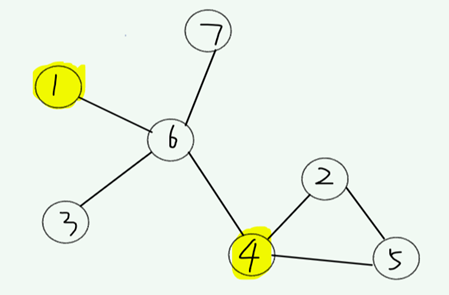此时解向量x={0,1,0,0,1,0,0,0}表示1,4号顶点此时加入解集合 邻接点数组c={0,0,1,0,0,1,2,0}表示2,5,6号顶点与U集合({1,4})直接相连，且6号顶点与2个U中顶点直接相连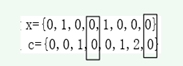满足x[j]==0&&c[j]==0(j从1到7)，不是完全覆盖 从图中可以看出不是一种完全覆盖。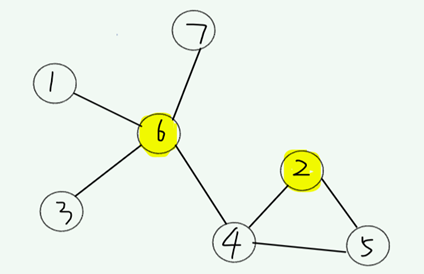解向量x={0,0,1,0,0,0,1,0} 表示2,6号顶点加入解集合 邻接点数组c={0,1,0,1,2,1,0,1} 表示1,3,7,4,5号顶点与U集合({2,6})直接相连，且4号顶点与2个U中顶点直接相连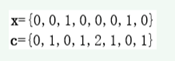不满足 x[j]==0&&c[j]==0(j从1到7), 是完全覆盖 从图中可以看出是一种完全覆盖，但是顶点的权值之和不一定最小。
解空间树：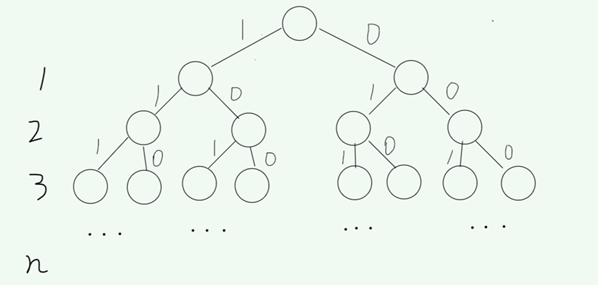每个顶点都有两种选择，加入U集合或者不加入U集合，显然进入右子树的选择一定是比进入左子树的选择所得的权值和小，但是进入右子树不一定满足顶点覆盖，所有当试探完左子树的结果后，还需进入右子树进行测试。
将每个试探的解向量加入到顶点权值小优先的优先队列，每次取出堆顶的解向量判断能否满足完全覆盖，如果能则得出最小权覆盖的解，因为堆顶解向量满足顶点权值和最小的原则，如果不能则继续取堆顶解向量判断。
优先队列存储空间示意图：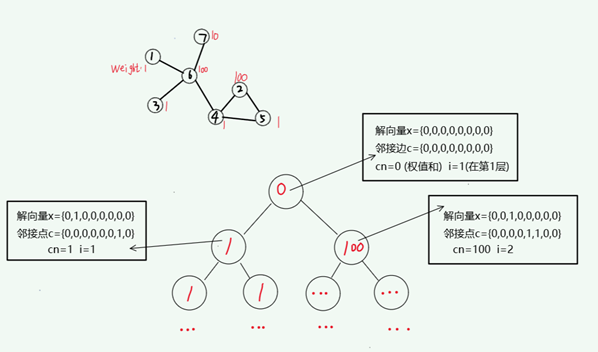图示为优先队列结点中存储的顶点权值和及其对应的解向量与邻接点数组。
——关于源代码应该都能搜到，所以这里就不再赘述了。
展开全文• 算法设计与分析第六章算法实现题第二题： ...将计算出的最小权顶点覆盖的顶点权之和以及最优输出到文件output.txt.文件第1行是最小权顶点覆盖顶点权之和;第2行是最优解xi,1≤i≤n,xi=0表示顶点i不在最小权顶点覆盖中.
• #include<iostream> using namespace std; int bestw=100000; int n,m; int tw=0; int connect; int w; int cover_in; int cover(int *cover_in){... cout最小权顶点覆盖："; return 0; } 实例：
#include<iostream>
using namespace std;
int bestw=100000;
int n,m;
int tw=0;
int connect;
int w;
int cover_in;
int cover(int *cover_in){
int cover_all;
for(int i=0;i<n;i++){
cover_all[i]=0;
}
for(int i=0;i<n;i++){
if(cover_in[i]==1){
cover_all[i]=1;
for(int j=0;j<n;j++){
if(connect[i][j]&&(cover_all[j]==0)){
cover_all[j]=2;
}
}
}
}
int count=0;
for(int i=0;i<n;i++){
if(cover_all[i]>0){
count++;
}
}
if(count==n){
return 1;
}
return 0;
}
int search(int level,int isLeft,int *cover_in){
if(level==0){
search(level+1,1,cover_in);
search(level+1,0,cover_in);
}
else if(level>n){
if(cover(cover_in)){
if(tw<bestw){
for(int i=0;i<n;i++){
cout<<cover_in[i]<<" ";
}
cout<<tw<<endl;
bestw=tw;
}
}
}
else{
if(isLeft){
cover_in[level-1]=1;
tw=tw+w[level-1];
search(level+1,1,cover_in);
search(level+1,0,cover_in);
cover_in[level-1]=0;
tw=tw-w[level-1];
}
else{
search(level+1,1,cover_in);
search(level+1,0,cover_in);
}
}
}
int main(){
int tempx,tempy;
cout<<"请输入顶点数和边数："<<endl;
cin>>n>>m;
cout<<"连接边："<<endl;
for(int i=0;i<m;i++){
int x,y;
cin>>x>>y;
connect[x-1][y-1]=1;
connect[y-1][x-1]=1;
}
cout<<"请输入顶点的权值："<<endl;
for(int i=0;i<n;i++){
cin>>w[i];
}
search(0,0,cover_in);
cout<<"最小权顶点覆盖："<<bestw;
return 0;
}

实例：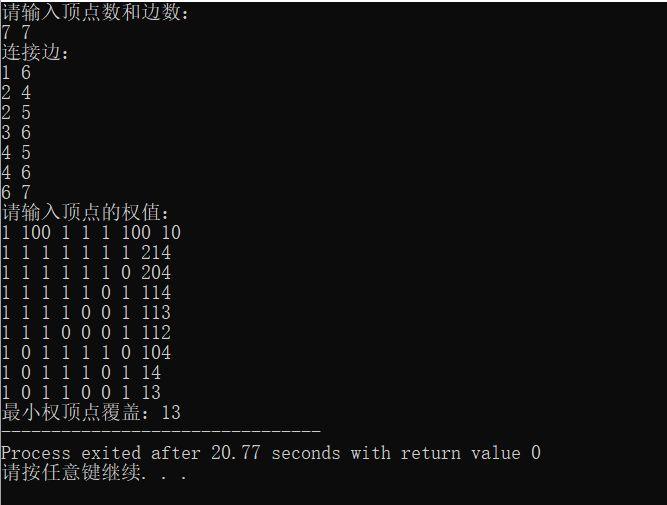展开全文• 问题描述
问题描述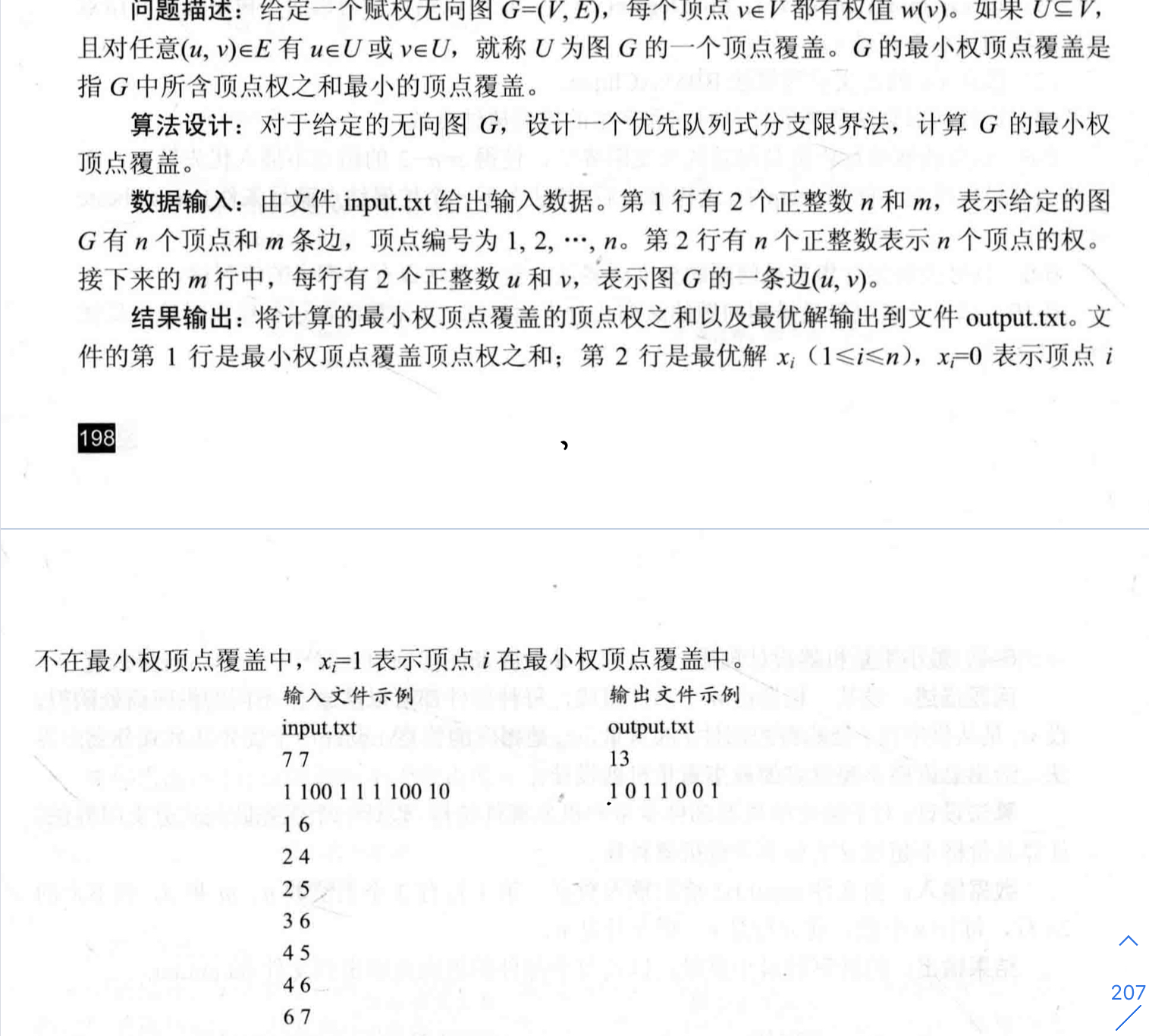题目分析
我们先简明介绍一下题目，我们仍然需要将所有点分成U、V两个集合。什么叫做顶点覆盖呢？即为V 集合中的每个点，至少与一个U集合中的点直接相连。如图所示（红色点表示U集合中的点）：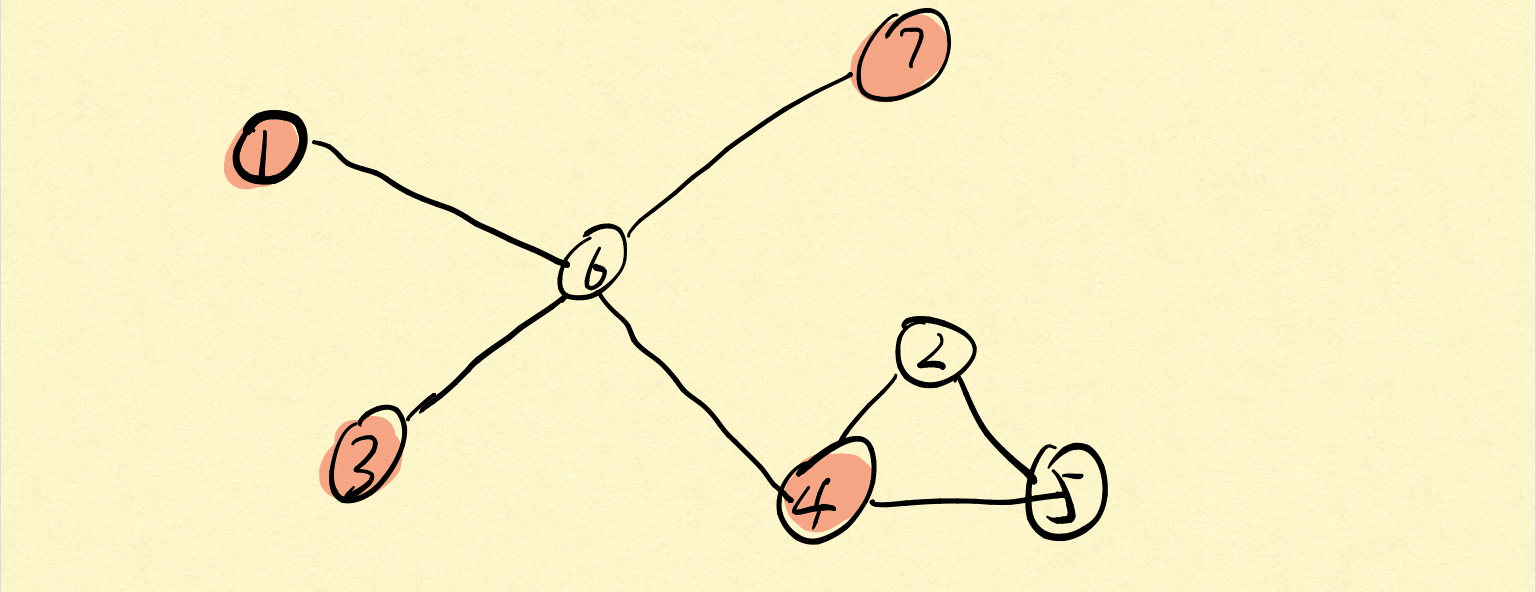我们可以看到V集合中的顶点2、5、6，都与至少一个U集合中的顶点直接相连。反而如果按照下图分配则不满足条件：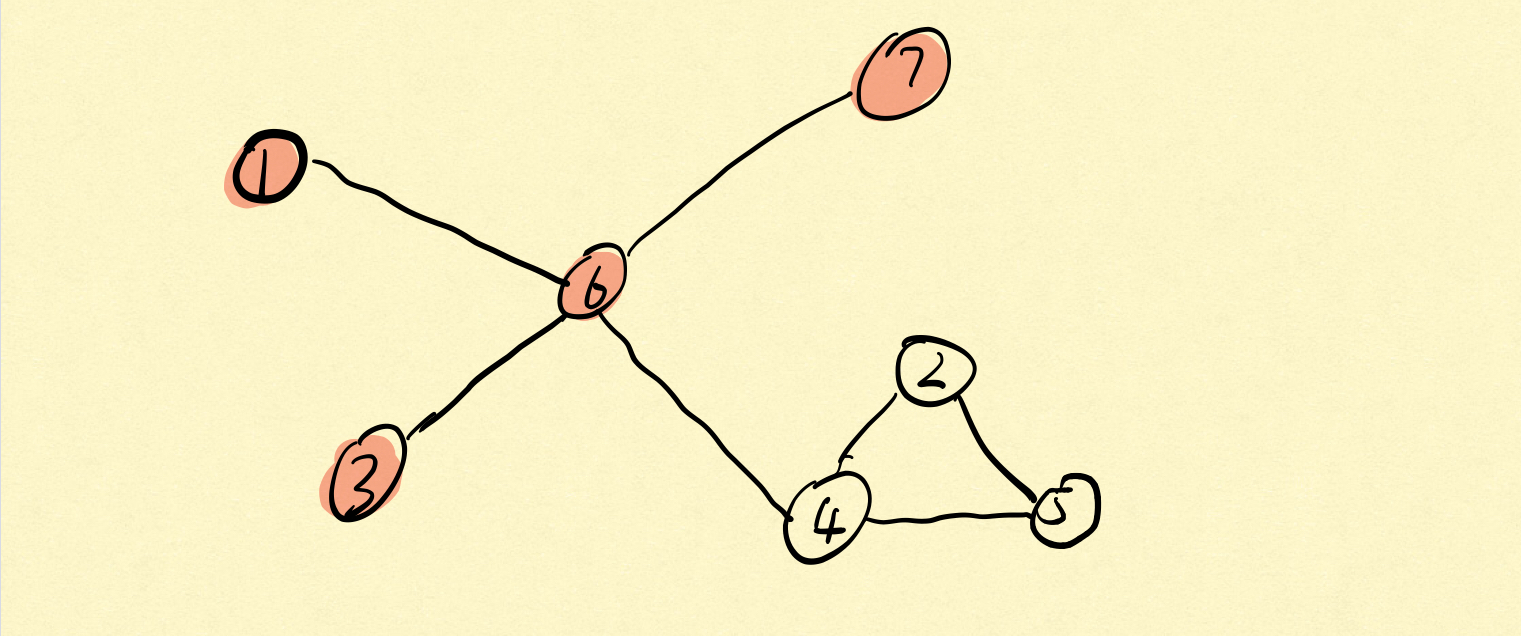图中V集合的顶点2、5并没有U集合中的点与其直接相连，所以不是一种顶点覆盖。
那我们应该如何判断图是否被覆盖了呢？可以开辟一个数组c，如果c[j]==0，则表示U集合中没有任何一个顶点与其直接相连。 优先级 说完如何判断是否覆盖之后，我们来确定一下优先级。由于所有顶点都是带权的，我们的目的也是找到最小权覆盖，所以我们可以直接用权重作为优先级建立一个最小堆，从而实现优先队列。 界限函数 我们找的是最小点权，无法使用界限函数来对右孩子进行约束，因为如果当前结点不加入U集合中(即走右孩子路径)，一定点权和更小，但是不一定会覆盖，所以不经过判断，我们也要将右孩子加入到队列中。 将活结点加入队列中 将活结点加入队列时，要对点的优先级、结果向量以及cover数组进行更新。
代码
#include <iostream>
#include <queue>
using namespace std;
class HeapNode
{
friend class VC;//求解最小权覆盖问题的类,融合了所有函数和所需的参数
public:
operator ()(int x,int y) const{return x < y;}//定义优先级
private:
int i,cn,*x,*c;//i表示结点序号,cn表示当前权重,x表示结果数组,c数组表示此时是否有一点i属于U,且i与j相连,如果有,则c[j]!=0
};
//解最小权顶点覆盖大类
class VC
{
friend MinCover(int **,int [],int);
private:
void BBVC();
bool cover(HeapNode E);//判断图是否已经被全部覆盖了
void AddLiveNode(priority_queue<HeapNode> &H,HeapNode E,int cn,int i,bool ch);
int **a,n,*w,*bestx,bestn;//邻接矩阵,节点数目,每个点的权重,结果向量,最优解
};
void VC::BBVC()
{
priority_queue<HeapNode> H(100000);
HeapNode E//扩展结点
E.x = new int [n+1];//开辟结果向量
E.c = new int [n+1];//开辟一数组,用于判断图是否被完全覆盖
for(int j = 1;j <= n;j++)
{
E.x[j] = E.c[j] = 0;
}
int i = 1,cn = 0;//初始化当前点权总和为0
while(true)
{
if(i > n)
{
if(cover(E))
{
for(int j = 1;j <= n;j++)
bestx[j]=E.x[j];
bestn = cn;
break;
}
}
else
{
if(!cover(E))//如果当前没有完全覆盖,就将这个点加入到U集合中
}
if(H.size()==0)
break;
E = H.top();
H.pop();
cn = E.cn;
i = E.i + 1;
}
}
//判断图是否完全覆盖
bool VC::cover(HeapNode E)
{
for(int j = 1;j <= n;j++)
{
if(E.x[j]==0 && E.c[j]==0)//如果此时j结点既不是U中的点,而且也没有U中的点与其相连,则至少这个点未被覆盖
{
return false;
}
}
return true;
}
void VC::AddLiveNode(priority_queue<HeapNode> &H,HeapNode E,int cn,int i,bool ch)
{
HeapNode N;//创建一个新的堆结点
N.x = new int [n+1];
N.c = new int [n+1];
for(int j = 1;j <= n;j++)
{
N.x[j] = E.x[j];
N.c[j] = E.c[j];
}
N.x[i] = ch;
if(ch)
{
N.cn = cn + w[i];//此时i要加入集合U,所以其权重应该加上cn
for(int j = 1;j <= n;j++)
{
if(a[i][j])
N.c[j]++;//表明此时对于结点j来说,有一节点i属于U与其连接,表明这个点被覆盖了
}
}
else
N.cn = cn;
N.i = i;
H.push(N);
}
//MinCover完成最小覆盖计算
int MinCover(int **a,int v[],int n)//v表示的是结点权重数组
{
VC Y;
Y.w = new int [n+1];
for(int j = 1;j <= n;j++)
Y.w[j] = v[j];
Y.a = a;
Y.n = n;
Y.bestx = v;
Y.BBVC();
return Y.bestn;
}
//主函数
int main()
{
int n,e,u,v;//结点数,边数,u,v为结点编号
cin>>n>>e;
int a[n+1][n+1];
for(int i = 0;i <= n;i++)
{
for(int j = 0;j <= n;j++)
{
a[i][j] = 0;//初始化为0
}
}
p = new int [n+1];//定义结果向量
for(int i = 1;i <= e;i++)
{
cin>>u>>v;
a[u][v] = 1;
a[v][u] = 1;
}
cout<<MinCover(a,p,n)<<endl;
for(int i = 1;i<=n;i++)
cout<<p[i]<<" ";
cout<<endl;
return 0;
}

总结
我们可以看到，判断是否覆盖需要O(n)的时间复杂度，活结点入队需要O(n)的时间复杂度。所以在BBVC函数中，时间复杂度应该为O(n3)（**个人理解，未必正确：while循环的时间复杂度与节点数目有关，所以是n级别的，在if(!cover(E))中，判断的时间复杂度为O(n)，内部的活结点入队操作时间复杂度为O(n)，所以BBVC的时间复杂度为O(n3)**）。在主函数中，MinCover与BBVC的时间复杂度相同，我们还需要输入邻接矩阵的边，所以时间复杂度为O(n^3+e)。（个人理解，如果有不同想法欢迎指出，谢谢）
展开全文• 众数问题 算法分析 题意描述 输入一个大小为n的有序数组，求该数组中出现次数最多的数，及其出现的次数，注：当有多个数出现次数最多时，只输出一个。 算法过程描述 假设我们先求出中位数的重数，再求出中位数往左往...
• 最小权顶点覆盖问题 问题描述： 给定一个赋权无向图G=(V,E)，每个顶点v∈V都有一个权值w(v)。如果 ，且对任意(u,v)∈E有u∈U或v∈U，就称U为图G的一个顶点覆盖。G的最小权顶点覆盖是指G中所含顶点权之和最小的顶点...
• 文件第2行是最优解Xi.1≤i≤n,Xi=0表示顶点i不在最小权顶点覆盖中,Xi=1表示顶点i在最小权顶点覆盖中. 输入文件示例 输出文件示例 Input.txt output.txt 7 7 13 1 100 1 1 1 100 10 1 0 1 1 0 0 1 1 6 2 4...
• 一个有效的本地搜索框架，可解决最小加权顶点覆盖问题研究论文
•研究论文
• 在用贪心法解决最小顶点覆盖问题中，它贪的是覆盖的边最多的顶点。用邻接矩阵存储无向图，矩阵中每行的和即为顶点的出度。出度最多的顶点即为最优量度标准。 最小顶点覆盖其一般特性： (1)找覆盖边最多的顶点 (2)已...算法
• 最小覆盖 = 最大独立集 = |V| - 最大匹配数 这个是在原图是二分图上进行的 最小路径覆盖最小覆盖不同，不要求给的图是二分图，而是要求是N x N的有向图，不能有环，然后根据原图构造二分图，构造方法是将点...
•算法
• 最小覆盖 = 最大独立集 = |V| - 最大匹配数 这个是在原图是二分图上进行的 最小路径覆盖最小覆盖不同，不要求给的图是二分图，而是要求是N x N的有向图，不能有环，然后根据原图构造二分图，构造方法是...
• 最小顶点覆盖：实质是个点集，点集里面的点能覆盖所有的边，最小点覆盖就是满足这个要求的点集中点数最小的那个 2、最大独立集 = 顶点个数V - 最大匹配数 最大独立集：实质是个点集，点集里面的点互相之间不连着，...
• 显然问题就转化为求最小顶点覆盖 又因为二分图中 最小顶点覆盖=最大匹配 so: #include #include #include #include #include #include #include #include #include #include #include ...HDU 二分匹配
• 最小权顶点覆盖问题 给 定一个赋权无向图G=(V,E)，每个顶点v∈V都有一个权值w(v)。如果U包含于V，且对于(u,v)∈E 有u∈U 且v∈V-U，则有v∈K.如：U = {1}, 若有边（1，2）， 则有2属于K. 若有集合U包含于V使得U + K......